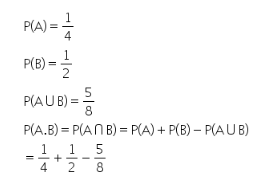# P(A)= 1/4 , P(B)= 1/2, P(AUB)= 5/8 then p(AB) is equal to?

P(A)= 1/4 , P(B)= 1/2, P(AUB)= 5/8 then p(AB) is equal to ?